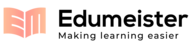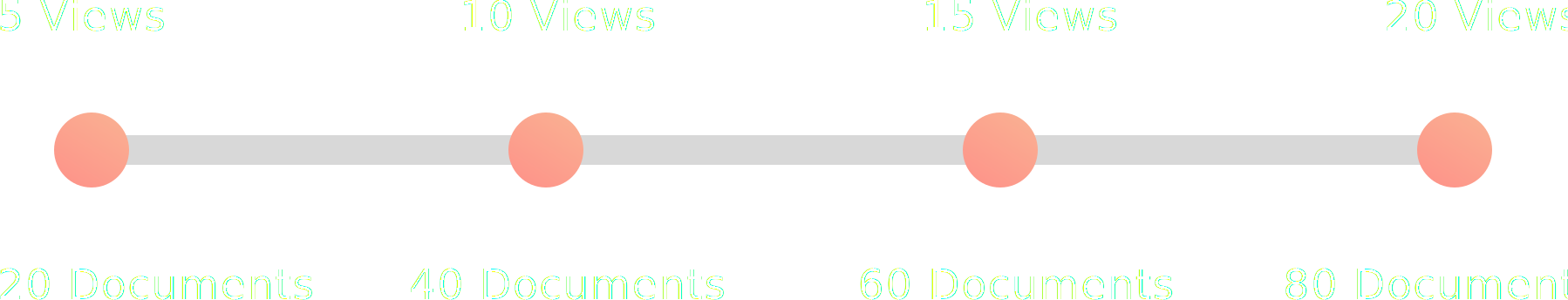## Page 1 of 13 MGT425 MCQ TB CH8-12 MGT425 – Spreadsshowing page 8-9 out of 13Page
8
of
13
MGT425 MCQ TB CH8-12
6.
Refer to Figure 11.1. According to the solution presented in the spreadsheet
a.
Project 1 gets implemented, but all the other projects do not
b.
Project 2 gets implemented, but all the other projects do not
c.
Project 3 gets implemented, but all the other projects do not
d.
Project 4 gets implemented, but all the other projects do not
e.
Project 5 gets implemented, but all the other projects do not
7.
Refer to Figure 11.1. Adding a binary constraint on the decisions:
a.
Will not change the solution
b.
Will improve (increase) the Total Value for the Objective
c.
Will deteriorate (decrease) the Total Value for the Objective
d.
Will improve (increase) the values of the constraints
e.
Will deteriorate (decrease) the values of the constraints
Figure 11.2. Capital Budgeting with a Contingency Constraint
8.
Refer to Figure 11.2. According to the solution, the projects that get implemented are:
a.
1 and 2
b.
2 and 4
c.
2 and 5
d.
3 and 5
e.
4 and 5
9.
Refer to Figure 11.2. What is the relationship between the projects?
a.
Project 5 is contingent upon project 3
b.
Project 5 is contingent upon project 2
c.
Project 4 is contingent upon project 3
d.
Project 1 is contingent upon project 4
e.
Cannot be determined from the information provided
Page
9
of
13
MGT425 MCQ TB CH8-12
10.
The use of nonlinear solver with integer constraints:
a.
Provides the best solution
b.
Will always find global optimum
c.
Is problematic due to the fact that it can get stuck at a local optimum
d.
Is recommended for all problems
e.
Is as effective as a linear solver
Chapter 12:
1.
An evolutionary solver:
a.
Always provides the optimal solution
b.
Is preferable for use on the models containing nonsmooth functions
c.
Always provides the same solution as a nonlinear solver
d.
Always provides the same solution as a linear solver
e.
None of the above
2.
The most flexible type of solver (in terms of the objective function) is:
a.
A linear solver
b.
A nonlinear solver
c.
An evolutionary solver
d.
All of the above
e.
None of the above
3.
If an objective function exhibits gaps
or sharp corners, the most appropriate type of solver to find
an optimal solution is:
a.
A linear solver
b.
A nonlinear solver
c.
An evolutionary solver
d.
All of the above
e.
None of the above
4.
If an objective function includes MAX() function, the most appropriate type of solver to find an
optimal solution is:
a.
A linear solver
b.
A nonlinear solver
c.
An evolutionary solver
d.
All of the above
e.
None of the above
5.
If an objective function includes the sum of squared deviations, the most appropriate type of
solver
to find an optimal solution is:
a.
A linear solver
b.
A nonlinear solver
c.
An evolutionary solver
d.
All of the above
e.
None of the above